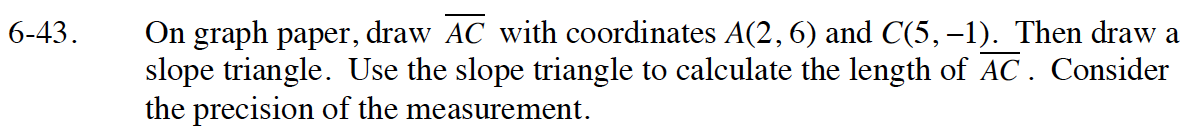Home > GB8I > Chapter cc46 > Lesson cc46.1.4 > Problem6-43

6-43.

On graph paper, draw AC with coordinates A(2, 6) and C(5, −1). Then draw a slope triangle. Use the slope triangle to calculate the length of AC. Consider the precision of the measurement. Homework Help ✎One leg is 3 units long, the other leg is 7 units long. You will need the Pythagorean Theorem.
Review the Math Notes box in section 4.1.1 if you need further help with the Pythagorean Theorem.